Test: Fourier Analysis of Discrete Time Signals

# Test: Fourier Analysis of Discrete Time Signals

Test Description

## 10 Questions MCQ Test GATE Electrical Engineering (EE) 2023 Mock Test Series | Test: Fourier Analysis of Discrete Time Signals

Test: Fourier Analysis of Discrete Time Signals for Electronics and Communication Engineering (ECE) 2023 is part of GATE Electrical Engineering (EE) 2023 Mock Test Series preparation. The Test: Fourier Analysis of Discrete Time Signals questions and answers have been prepared according to the Electronics and Communication Engineering (ECE) exam syllabus.The Test: Fourier Analysis of Discrete Time Signals MCQs are made for Electronics and Communication Engineering (ECE) 2023 Exam. Find important definitions, questions, notes, meanings, examples, exercises, MCQs and online tests for Test: Fourier Analysis of Discrete Time Signals below.
Solutions of Test: Fourier Analysis of Discrete Time Signals questions in English are available as part of our GATE Electrical Engineering (EE) 2023 Mock Test Series for Electronics and Communication Engineering (ECE) & Test: Fourier Analysis of Discrete Time Signals solutions in Hindi for GATE Electrical Engineering (EE) 2023 Mock Test Series course. Download more important topics, notes, lectures and mock test series for Electronics and Communication Engineering (ECE) Exam by signing up for free. Attempt Test: Fourier Analysis of Discrete Time Signals | 10 questions in 30 minutes | Mock test for Electronics and Communication Engineering (ECE) preparation | Free important questions MCQ to study GATE Electrical Engineering (EE) 2023 Mock Test Series for Electronics and Communication Engineering (ECE) Exam | Download free PDF with solutions
 1 Crore+ students have signed up on EduRev. Have you?
Test: Fourier Analysis of Discrete Time Signals - Question 1

### The discrete timefourier transform for the given signal x[n] - u[n] is

Detailed Solution for Test: Fourier Analysis of Discrete Time Signals - Question 1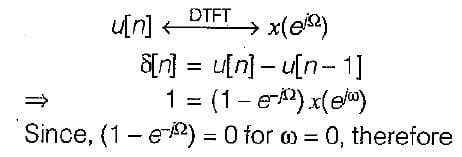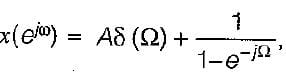where A is constant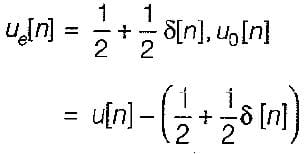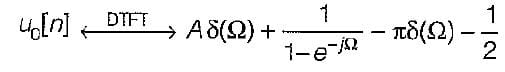The Fourier transform of odd real sequences must be purely imagenary, thus A = π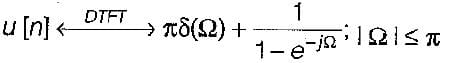Test: Fourier Analysis of Discrete Time Signals - Question 2

### The discrete time Fourier coefficients x[k] of the signal x [n ] =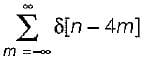Detailed Solution for Test: Fourier Analysis of Discrete Time Signals - Question 2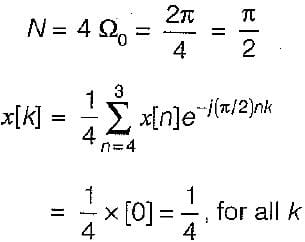Test: Fourier Analysis of Discrete Time Signals - Question 3

### The zero state respone y(k) for input f(k) = (0.8)k u(k) is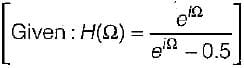Detailed Solution for Test: Fourier Analysis of Discrete Time Signals - Question 3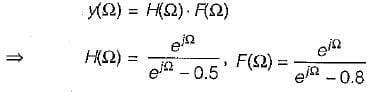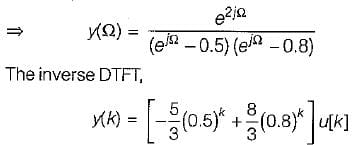Test: Fourier Analysis of Discrete Time Signals - Question 4

Consider discrete time sequence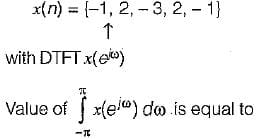Detailed Solution for Test: Fourier Analysis of Discrete Time Signals - Question 4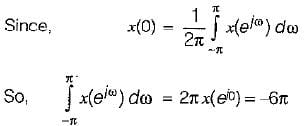Test: Fourier Analysis of Discrete Time Signals - Question 5

Consider a signal x(n) with following factors:
1. x(n) is real and even signal
2. The period of x(n) is N = 10
3. x(11) = 5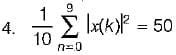The signal x(n) is

Detailed Solution for Test: Fourier Analysis of Discrete Time Signals - Question 5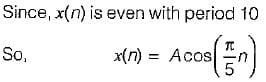Test: Fourier Analysis of Discrete Time Signals - Question 6

Consider a discrete time signal x(n) = {-1, 2, -3, 2, -1} value of ∠x(e) is equal to

Test: Fourier Analysis of Discrete Time Signals - Question 7

A low pass filter with impulse response h1(n) has spectrum H1 (e) shown below.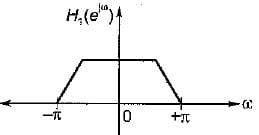Here only one period has been shown by reversing every second sign of h1(n) a new filter having impulse response  h2(t) is created. The spectrum of H2(e) is given by

Test: Fourier Analysis of Discrete Time Signals - Question 8

A red signal x[n] with Fourier transform x(e) has following property:
1. x[n] = 0 for, n > 0
2. x [ 0] > 0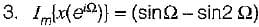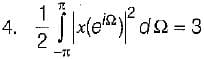The signal x[n] is

Test: Fourier Analysis of Discrete Time Signals - Question 9

A causal and stable LTI system has the property that,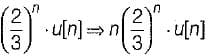The frequency response H(e) for this system is

Test: Fourier Analysis of Discrete Time Signals - Question 10

A 5-point sequence x[n] is given as 4 [- 3] = 1, x[ - 2] = 1, x[ -1 ] = 0 . x = 5 , x - 1
Let x(e) denote the discrete time fourier transform of x[n].
The value of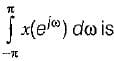## GATE Electrical Engineering (EE) 2023 Mock Test Series

22 docs|274 tests
Information about Test: Fourier Analysis of Discrete Time Signals Page
In this test you can find the Exam questions for Test: Fourier Analysis of Discrete Time Signals solved & explained in the simplest way possible. Besides giving Questions and answers for Test: Fourier Analysis of Discrete Time Signals, EduRev gives you an ample number of Online tests for practice

## GATE Electrical Engineering (EE) 2023 Mock Test Series

22 docs|274 tests(Scan QR code)